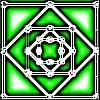#### You may also like### Homes

Six new homes are being built! They can be detached, semi-detached or terraced houses. How many different combinations of these can you find?### Number Squares

Start with four numbers at the corners of a square and put the total of two corners in the middle of that side. Keep going... Can you estimate what the size of the last four numbers will be?### I'm Eight

Find a great variety of ways of asking questions which make 8.

# Arranging Additions and Sorting Subtractions

##### Age 5 to 7Challenge Level

Well done to everyone who ordered their calculations and thought carefully about the reasoning behind their answers.

In the first part of the problem we asked you to order four addition calculations. Jasmine, from Meavy Church of England School, sent in this list:

42 + 3 = 45 (Easiest because you are only adding one digit)

42 + 18 = 60 (Second easiest because you can add up the tens 40 + 10 +10)

42 + 47 = 89 (Third easiest because you can add up tens and ones easily, 40 + 40 + 9)

42 + 39 = 81 (Hardest because 9 + 2 = 11 which goes over the tens, 40 + 30 + 11)

Look careful at Jasmine's reasoning for her answers. Do you agree with her ordering, or would you have ordered the calculations another way?

Minha, who is from St Michael's International School in Kobe, Japan sent in her list too. What's the same about Minha's and Jasmine's lists? What's different about them?

My answer is 42+39, 42+18, 42+47, 42+3.

42 + 39 is the hardest because when I calculate 9+2, It is 11. So you have to carry the 10.

And the second hardest is 42+18 is 60, for 2+8 is 10 so you have to carry the 10, but it is easier as there are no ones to remember.

And the next hardest is 42+47 because it is a little bit easier to do, because 7+2 is 9 and 40 plus 40 is eighty so it is a little bit easier to calculate it.

Finally, 42 plus 3 is easiest to calculate because it doesn't need to put in a tens box. And we need to just add on 3 ones. And it is 45.

Simran, from Maurice Hawk School in the USA, sent in her list and shared her reasoning too:

42 + 3 is easiest to add because you are only adding digits in the ones place and they add up to less than 10, so there is no regrouping.

42 + 47 is easy because 2 + 7 in the ones place makes 9. 40 and 40 in the tens place makes 80. Then you add 9 and 80, the total is 89. Regrouping in not required.

42 + 18 is also easy to add. 2 and 8 in the ones place of both numbers add up to 10. 40 and 10 in the tens place add up to 50. Adding 10 to any number is easy. So, the total is 60.

42 + 39 is the hardest because of regrouping. First, I added 2 and 9 in the ones place which is 11. Then, I added 40 + 30 which is 70. Finally, I added 70 + 11 which gives a total of 81.

Simran also shared another way to calculate 42 + 18:

There is another way to solve this: First I take 2 from 42 and add it to 18 to make 20. Then add 40 to 20 and the total is 60.

The second part of this problem asks you to order four subtraction calculations. Both Jasmine and Simran shared their ideas for this part of the problem too.

Let's begin by looking at Jasmine's solution:

26 - 10 is the easiest because 10 is in the tens so is 26 so you take 10 away from 26 and the answer is 16.

26 - 3 is the 2nd easiest because 3 is a unit so you can do this on your fingers count backwards from 26 three times and you get 23.

26 - 7 this is the 3rd easiest because if you count backward from 26 seven times you will get 19.

26 - 18 is the hardest because if I had 26 apples and I ate 18 I would be left with 8 apples.

Now let's look at Simran's list. What do you notice about her solution?

26 - 3 is the easiest because I know that 6 is a double of 3. So, when I subtract 3 from 26, the difference is 23.

26 - 10 is also very easy. You can think about the 100s chart. The number above is 10 less and the number below is 10 more on the hundreds chart. Think about the square right above 26 and you get 16.

26 - 7 is not hard. First, I subtracted 6 from 20 which gives the difference of 20, then I subtracted 1 more and I get 19.

26 - 18 is the hardest because you have to do regrouping. First, I subtracted 8 from 16 which gives a difference of 8, then I had 10 left in the tens place. Then, I subtracted 10 from 10 (in the tens place), so the difference was 8. Another way : First I add 2 to 18 to make it 20 because it is very easy to subtract 20. Subtracting 20 from 26 gives a difference of 6 but because I subtracted two extra numbers, we have to add them back to 6. So, the difference of 26 - 18 is 8.

Many thanks to Jasmine, Simran and Minha for sharing their reasoning behind their answers.

Having read through their answers, I'm wondering if you could write your own lists of calculations and share the reasons behind their ordering?